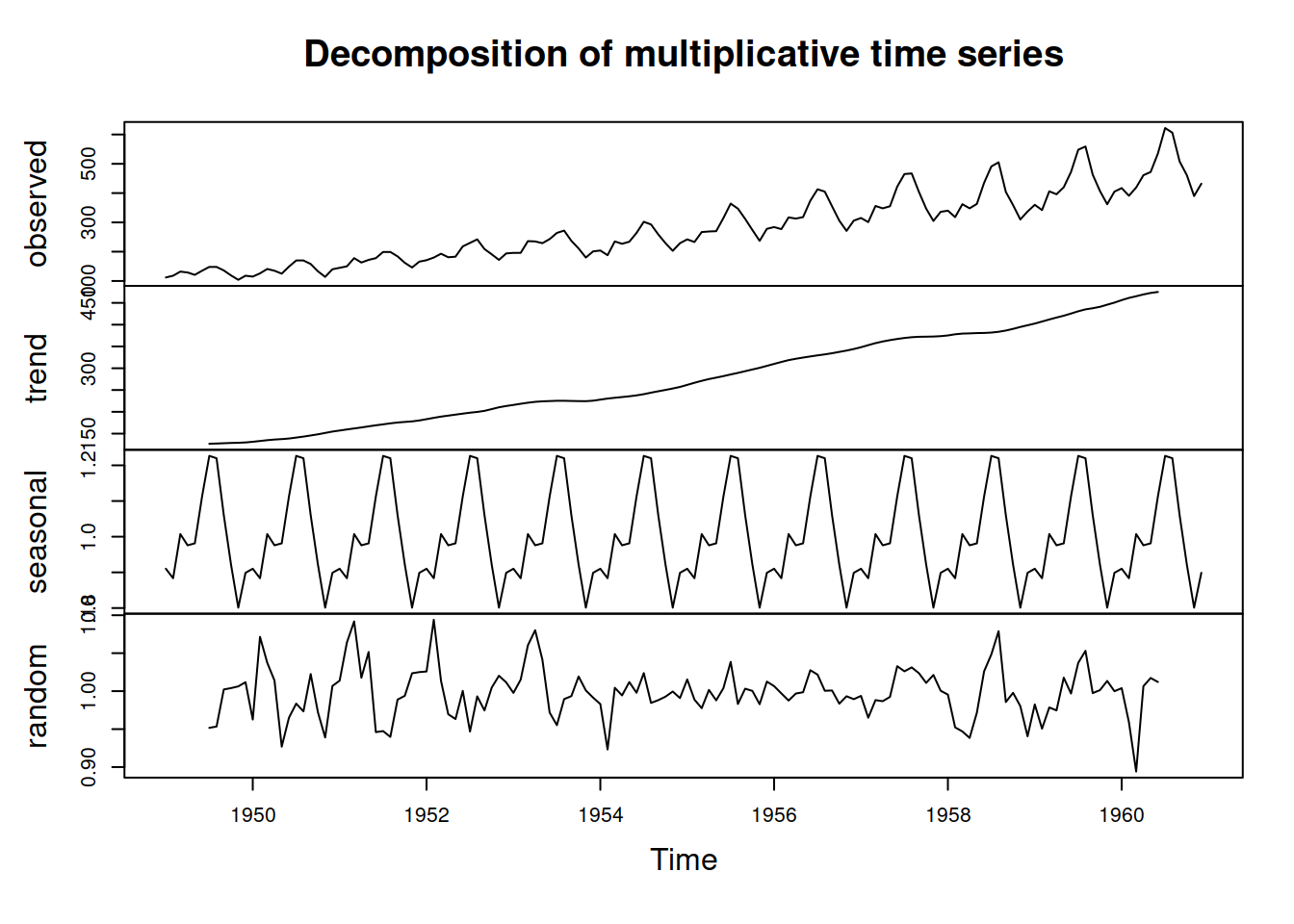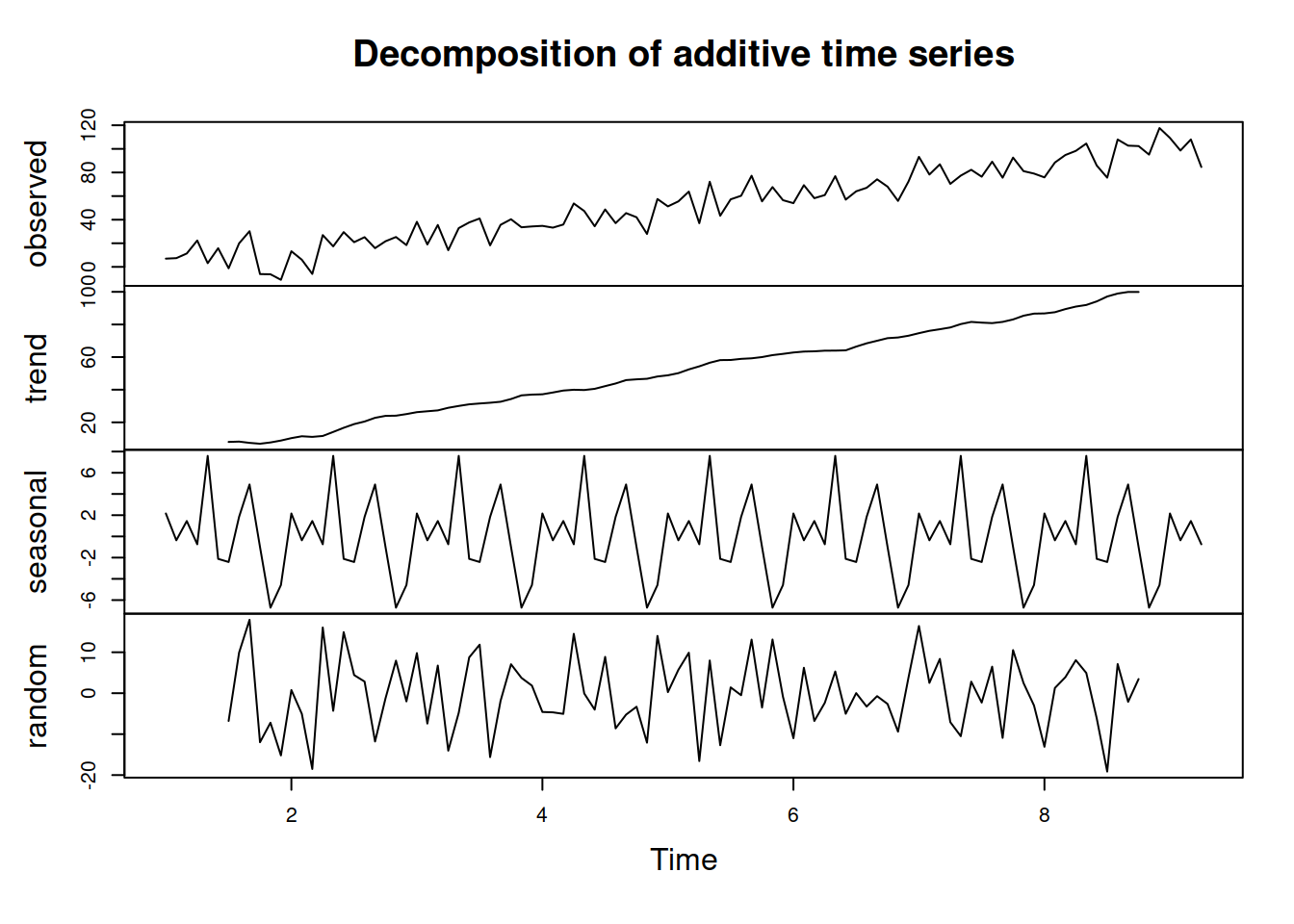This book is in Open Review. I want your feedback to make the book better for you and other readers. To add your annotation, select some text and then click the on the pop-up menu. To see the annotations of others, click the button in the upper right hand corner of the page

## 4.2 Classical Seasonal Decomposition

### 4.2.1 How to do?

One of the classical textbook methods for decomposing the time series into unobservable components is called "Classical Seasonal Decomposition" (Warren M. Persons 1919). It assumes either a pure additive or pure multiplicative model, it is done using centred moving averages and is focused on approximation, not on forecasting. The idea of the method can be summarised in the following steps:

1. Decide, which of the models to use based on the type of seasonality in the data: additive (4.1) or multiplicative (4.2)
2. Smooth the data using centred moving average (CMA) of the order equal to the periodicity of the data $$m$$. If $$m$$ is the odd number then the formula is the following: $\begin{equation} d_t = \frac{1}{m}\sum_{i=-(m-1)/2}^{(m-1)/2} y_{t+i}, \tag{4.4} \end{equation}$ which means that, for example, the value on Thursday is the average of values from Monday to Sunday. If $$m$$ is the even number, then a different weighting scheme is typically used, involving the inclusion of additional value: $\begin{equation} d_t = \frac{1}{m}\left(\frac{1}{2}\left(y_{t+(m-1)/2}+y_{t-(m-1)/2}\right) + \sum_{i=-(m-2)/2}^{(m-2)/2} y_{t+i}\right), \tag{4.5} \end{equation}$ which means, for example, that we take a half of December of the previous year and half of December of this year in order to calculate the centred moving average in June. The values $$d_t$$ are placed in the middle of the windows, going through the series (e.g. on Thursday the average will contain values from Monday to Sunday).

The resulting series corresponds to the deseasonalised data. Indeed, when we, for instance, take an average values of the sales in a year, we automatically remove the potential seasonality, which can be observed individually in each month. A drawback from using CMA is that this way we inevitably loose $$\frac{m}{2}$$ observations from the head and from the tail of the series.

In R, ma() function from forecast package implements CMA.

1. De-trend the data:
• For the additive decomposition this is done using: $${y^\prime}_t = y_t - d_t$$;
• For the multiplicative one, it is: $${y^\prime}_t = \frac{y_t}{d_t}$$;
1. If the data is seasonal, then the average value for each period is calculated based on the de-trended series. e.g. we produce average seasonal indices for each January, February, etc. This will give us the set of seasonal indices $$s_t$$;
2. Calculate the residuals based on what you assume in the model:
• additive seasonality: $$e_t = y_t - d_t - s_t$$;
• multiplicative seasonality: $$e_t = \frac{y_t}{d_t s_t}$$;
• no seasonality: $$e_t = {y^\prime}_t$$.

Note that the functions in R typically allow selecting between additive and multiplicative seasonality only, there is no option for "none", so inevitably you will get the value of $$s_t$$ in the output, even if the data is not seasonal. Also, notice that the classical decomposition assumes that there is $$d_t$$ - deseasonalised series, but it does not make any further split of this variable into level $$l_t$$ and trend $$b_t$$.

### 4.2.2 A couple of examples

An example of the classical decomposition in R is the decompose() function from stats package. Here is an example with pure multiplicative model and AirPassengers data:

ourDecomposition <- decompose(AirPassengers, type="multiplicative")
plot(ourDecomposition)We can see that the function has smoothed the original series and produced the seasonal indices. Note that the trend component has gaps in the beginning and in the end. This is because the method relies on CMA, which results in loosing $$\frac{m}{2}$$ observations in those parts (as mentioned above). We can also notice that the error term still contains some seasonal elements, which is a downside of such a simple decomposition procedure. However, the lack of precision in this method is compensated by the simplicity and speed of calculation. Note again that the trend component in decompose() function is in fact $$d_t = l_{t}+b_{t}$$.

And here is an example of decomposition of the non-seasonal data (we assume pure additive model in this example):

y <- ts(c(1:100)+rnorm(100,0,10),frequency=12)
plot(ourDecomposition)As you can see, the original data has no seasonality in it, but the decomposition assumes that there is one and proceeds with the default approach, returning the seasonal component. This is expected, because with the time series decompositions you get what you ask for.

### 4.2.3 Other techniques and "Why bother?"

There are other decomposition techniques that do a similar split into Error-Trend-Seasonal components with different assumptions. The logic behind them is roughly the same: (1) smooth original series, (2) extract seasonal components, (3) smooth them out. The methods differ by the smoother they use (e.g. Bisquare function in LOESS instead of CMA), and in some cases the smoothing is done several times, to make sure that the components are split correctly.

There are many functions in R that implement seasonal decomposition, here are some of them:

• decomp() function from tsutils package does classical decomposition and fills in the tail and head of the smoothed trend with forecasts from exponential smoothing;
• stl() function from stats uses a different approach - seasonal decomposition by LOESS. It is an iterative algorithm that smoothes the states and allows them evolving over time. So, for example, the seasonal component in STL can change;
• mstl() from forecast package does the STL for data with several seasonalities;
• msdecompose() from smooth does a classical decomposition for multiple seasonal series.

Now the question is, "Why bother with the decomposition?". In my opinion, understanding the idea of decomposition helps in understanding ETS, which relies on it. From the practical point of view, it can be useful if you want to see, if there is a trend in the data and whether the residuals contain outliers or not. As mentioned above, the seasonality is forced in the decomposition, so it cannot tell you if the data is seasonal or not (I stress this out here, because many students think otherwise). Additionaly, when seasonality cannot be added to the model under consideration, decomposing the series, predicting the trend and then reseasonalising can be a viable a solution. Finally, the values from the decomposition can be used as starting points for the estimation of components in ETS or other dynamic models, relying on the error-trend-seasonality.

### References

Warren M. Persons. 1919. “General Considerations and Assumptions.” The Review of Economics and Statistics 1 (1): 5–107. doi:10.2307/1928754.